# Bayesian Statistical Methods

## Partial solutions

### Chapter 5: Model selection and diagnistics

Jump to probem: 1, 3, 5, 7, 9, 11

(1) Although other options are possible, we will use the unit information prior and evaluate methods using mean squared prediction error.

# Load the data

data(airquality)
Y     <- airquality$Ozone solar <- scale(airquality$Solar.R)
temp  <- scale(airquality$Temp) wind <- scale(airquality$Wind)
X1    <- cbind(1,solar)
X2    <- cbind(1,solar,temp,wind)

# Remove observations with missing data

miss  <- is.na(Y+rowSums(X2))
Y     <- Y[!miss]
X1    <- X1[!miss,]
X2    <- X2[!miss,]
n     <- length(Y)

# Split the data into five folds

fold  <- sample(1:5,n,replace=TRUE)
Yhat1 <- rep(NA,n)
Yhat2 <- rep(NA,n)
for(f in 1:5){
train <- which(fold!=f)
test  <- which(fold==f)
X1tr  <- X1[train,] # Extract training data
X2tr  <- X2[train,]
Ytr   <- Y[train]
c     <- length(Ytr)/(1+length(Ytr))

# Compute the posterior mean based on the training data (could use lm)
b1    <- c*solve(t(X1tr)%*%X1tr)%*%t(X1tr)%*%Ytr
b2    <- c*solve(t(X2tr)%*%X2tr)%*%t(X2tr)%*%Ytr

# Make predictions
Yhat1[test] <- X1[test,]%*%b1
Yhat2[test] <- X2[test,]%*%b2
}

# Compute MSE
MSE1 <- mean((Y-Yhat1)^2)
MSE2 <- mean((Y-Yhat2)^2)

MSE1;MSE2

##  992.8065

##  464.0511


Model 2 with all three predictors gives much smaller MSE and is thus preferred.

(3) Guessing and checking gives $$b=0.0006$$. The posterior is then $$\lambda|Y\sim\mbox{Gamma}(Y+a,N+b)$$ and since the prior probability of each hypothesis is equal, the Bayes factor is simply $$\mbox{Prob}(\lambda>1|Y)/\mbox{Prob}(\lambda<1|Y)$$, which is greater than 10 only for the last two cases.

#Verify this b gives prior prob 0.5
a <- 0.1
b <- 0.0006
pgamma(1,a,b)

##  0.5005538

# Load the data
N <- c(10,20,50,100)
Y <- c(12,24,60,120)

# Compute P(lambda>1|Y)
Pa <- 1-pgamma(1,Y+a,N+b)
Pa

##  0.7071244 0.7935365 0.9099061 0.9723693

# Compute and plot the Bayes factor
BF <- Pa/(1-Pa)
BF

##   2.414419  3.843470 10.099530 35.191662

plot(N,BF,xlab="N",ylab="Bayes factor")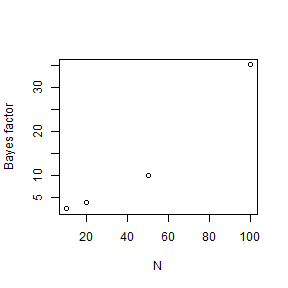(5)

library(rjags)

# Load the data
library(geoR)
gambia[1,]

##             x       y pos  age netuse treated green phc
## 1850 349631.3 1458055   1 1783      0       0 40.85   1

Y   <- gambia$pos X <- gambia[,4:8] X <- scale(X) # Fit logistic model mod <- textConnection("model{ for(i in 1:n){ Y[i] ~ dbern(pi[i]) logit(pi[i]) <- beta + X[i,1]*beta + X[i,2]*beta + X[i,3]*beta + X[i,4]*beta + X[i,5]*beta like[i] <- dbin(Y[i],pi[i],1) # For WAIC computation } for(j in 1:6){beta[j] ~ dnorm(0,0.01)} }") data <- list(Y=Y,X=X,n=length(Y)) model <- jags.model(mod,data = data, n.chains=2,quiet=TRUE) update(model, 5000, progress.bar="none") samps <- coda.samples(model, variable.names=c("like"), n.iter=20000, progress.bar="none") # Compute DIC DIC_logit <- dic.samples(model,n.iter=20000,progress.bar="none") # Compute WAIC like <- rbind(samps[],samps[]) # Combine the two chains fbar <- colMeans(like) Pw <- sum(apply(log(like),2,var)) WAIC_logit <- -2*sum(log(fbar))+2*Pw # Fit probit model mod <- textConnection("model{ for(i in 1:n){ Y[i] ~ dbern(pi[i]) probit(pi[i]) <- beta + X[i,1]*beta + X[i,2]*beta + X[i,3]*beta + X[i,4]*beta + X[i,5]*beta like[i] <- dbin(Y[i],pi[i],1) # For WAIC computation } for(j in 1:6){beta[j] ~ dnorm(0,0.01)} }") data <- list(Y=Y,X=X,n=length(Y)) model <- jags.model(mod,data = data, n.chains=2,quiet=TRUE) update(model, 5000, progress.bar="none") samps <- coda.samples(model, variable.names=c("like"), n.iter=20000, progress.bar="none") # Compute DIC DIC_probit <- dic.samples(model,n.iter=20000,progress.bar="none") # Compute WAIC like <- rbind(samps[],samps[]) # Combine the two chains fbar <- colMeans(like) Pw <- sum(apply(log(like),2,var)) WAIC_probit <- -2*sum(log(fbar))+2*Pw # Compare results DIC_logit;DIC_probit  ## Mean deviance: 2520 ## penalty 6.002 ## Penalized deviance: 2526  ## Mean deviance: 2521 ## penalty 5.972 ## Penalized deviance: 2527  WAIC_logit;WAIC_probit  ##  2525.647  ##  2527.103  Both criterion are very similar for both link functions, but slightly favor the logit link. (7) The intercept $$\beta_1$$ has uninformative Normal$$(0,10)$$. We fit model 2 with $$\beta_2 = 1 + \gamma\delta$$ where $$\gamma\sim\mbox{Bernoulli}(0.5)$$ and $$\delta\sim\mbox{Normal}(0,c^2)$$. This model has half its prior probability on each model because when $$\gamma=0$$ it reduces to model 1. The code below computes the posterior mean of $$\gamma$$, which is the posterior probability of model 2. This probability is computed for $$c\in\{0.5,1,2\}$$ to test for prior sensitivity. This range of log odds is quite diffuse on the probability scale.  library(rjags) # Load the data Y <- c(64,72,55,27,75,24,28,66,40,13) n <- c(75,95,63,39,83,26,41,82,54,16) q <- c(0.845,0.847,0.880,0.674,0.909, 0.898,0.770,0.801,0.802,0.875) X <- log(q/(1-q)) # Define the SSVS model: nba_model <- "model{ for(i in 1:10){ Y[i] ~ dbinom(pi[i],n[i]) logit(pi[i]) <- beta1 + beta2*X[i] } beta1 ~ dnorm(0,0.1) beta2 = 1+delta*gamma gamma ~ dbern(0.5) delta ~ dnorm(0,prec) }" # Conduct the analysis for various priors sds, c pri_sd <- c(0.5,1,2) for(c in pri_sd){ mod <- textConnection(nba_model) data <- list(Y=Y,X=X,n=n,prec=1/c^2) model <- jags.model(mod,data = data, n.chains=2,quiet=TRUE) update(model, 10000, progress.bar="none") samps <- coda.samples(model, variable.names=c("gamma"), n.iter=100000, thin=10,progress.bar="none") print(c) print(summary(samps)$statistics)
}

##  0.5
##           Mean             SD       Naive SE Time-series SE
##    0.301350000    0.458855865    0.003244601    0.003925080
##  1
##           Mean             SD       Naive SE Time-series SE
##    0.199100000    0.399333399    0.002823714    0.003789619
##  2
##           Mean             SD       Naive SE Time-series SE
##    0.107750000    0.310072161    0.002192541    0.002967053


As expected for this small dataset the results are somewhat sensitive to the prior distribution.

(9) We fit the multiple linear regression model $$Y_i\sim\mbox{Normal}(\beta_0+\sum_{j=1}^pX_{ij}\beta_j,\sigma^2)$$. The regression coefficients have SSVS priors $$\beta_j=\gamma_j\delta_j$$ where $$\gamma_j\sim\mbox{Bernoulli}(0.5)$$ and $$\delta_j\sim\mbox{Normal}(0,\tau^2)$$. The remaining priors are $$\beta_0\sim\mbox{Normal}(0,10^2)$$ and $$\sigma^2,\tau^2\sim\mbox{InvGamma}(0.1,0.1)$$.

library(rjags)
library(MASS)

# Load the data
names(Boston)

##   "crim"    "zn"      "indus"   "chas"    "nox"     "rm"      "age"
##   "dis"     "rad"     "tax"     "ptratio" "black"   "lstat"   "medv"

Y <- Boston[,14]
X <- scale(Boston[,1:13])

# Define the SSVS model:
SSVS_model <- "model{
for(i in 1:n){
Y[i] ~ dnorm(beta0 + inprod(X[i,],beta[]),tau1)
}
beta0 ~ dnorm(0,0.01)
for(j in 1:p){
beta[j]  = delta[j]*gamma[j]
gamma[j] ~ dbern(0.5)
delta[j] ~ dnorm(0,tau2)
}
tau1 ~ dgamma(0.1,0.1)
tau2 ~ dgamma(0.1,0.1)
}"

# Generate MCMC samples

mod <- textConnection(SSVS_model)
data  <- list(Y=Y,X=X,n=length(Y),p=ncol(X))
model <- jags.model(mod,data = data, n.chains=2,quiet=TRUE)
update(model, 10000, progress.bar="none")
samps <- coda.samples(model, variable.names=c("gamma"),
n.iter=50000, thin=1,progress.bar="none")

# Compute the posterior probability of each model
gamma <- rbind(samps[],samps[])
S     <- nrow(gamma)
model <- ""
for(j in 1:13){
temp  <- ifelse(gamma[,j]==1,colnames(X)[j],"")
model <- paste(model," ",temp)
}
most_common_model <- which.max(table(model))
colnames(gamma)   <-colnames(X)
marg_inc_probs    <- colMeans(gamma)

round(marg_inc_probs,2)

##    crim      zn   indus    chas     nox      rm     age     dis     rad
##    0.95    0.96    0.17    0.95    1.00    1.00    0.14    1.00    1.00
##     tax ptratio   black   lstat
##    0.97    1.00    0.98    1.00

 most_common_model

##    crim   zn      chas   nox   rm      dis   rad   tax   ptratio   black   lstat
##                                                                               83


The marginal inclusion probability exceeds 0.5 for all covariates except for “indus” and “age”. The most common model includes 11 predictors and excludes “indus” and “age”.

(11) To test whether the normality assumption is reasonable we use posterior predictive checks on the min, max and range of the data. Also, since this is a time series model we also check the min, max and range of the differences between consecutive observations.

# Load and plot the data
library(rjags)
Y <- as.vector(WWWusage)
plot(Y)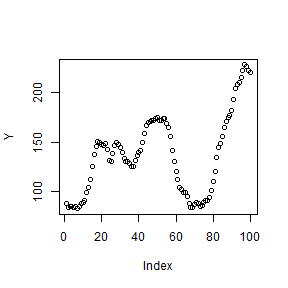hist(Y)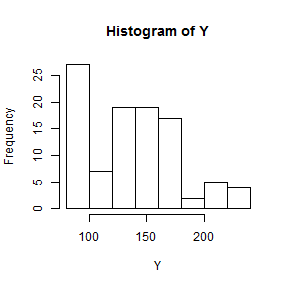hist(Y[2:100]-Y[1:99])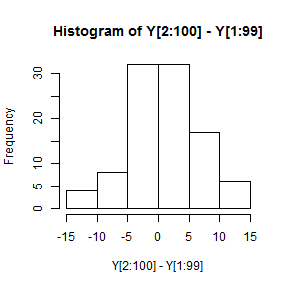# Define the AR2 model:
ar2_model <- "model{
for(t in 3:100){
Y[t]   ~ dnorm(mu[t],tau)
mu[t] <- beta + beta*Y[t-1] + beta*Y[t-2]
}
for(j in 1:3){beta[j] ~ dnorm(0,0.01)}
tau ~ dgamma(0.1,0.1)
sigma <- 1/sqrt(tau)
}"

# Run MCMC to generate posterior samples

mod <- textConnection(ar2_model)
data  <- list(Y=Y)
model <- jags.model(mod,data = data, n.chains=2,quiet=TRUE)
update(model, 10000, progress.bar="none")
samps <- coda.samples(model, variable.names=c("beta","sigma"),
n.iter=100000, thin=10,progress.bar="none")
samps <- rbind(samps[],samps[])
S     <- nrow(samps)

# Define the test statistics
test_stat <- function(Y){
diff <- Y[2:100]-Y[1:99]
d    <- c(min(Y),max(Y),max(Y)-min(Y),
min(diff),max(diff),max(diff)-min(diff))
return(d)}

# Compute the test statistics for the data and each sample
D0 <- test_stat(Y)

D <- matrix(0,S,6)
for(s in 1:S){
b   <- samps[s,1:3]
sig <- samps[s,4]
Yp  <- Y
for(t in 3:100){
Yp[t] <- b + b*Y[t-1] + b*Y[t-2]+rnorm(1,0,sig)
}
D[s,] <- test_stat(Yp)
}

# Plot the results
names <- c("Min Y","Max Y","Range Y",
"Min Diff","Max Diff","Range Diff")
for(j in 1:6){
pval <- mean(D[,j]>D0[j])
hist(D[,j],breaks=25,xlim=range(D[,j]) + 5*c(-1,1),
xlab=names[j],
main=paste0("Bayesian p-value = ",round(pval,2)))
abline(v=D0[j],lwd=2,col=2)
}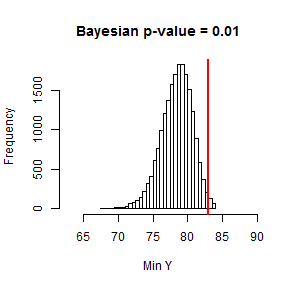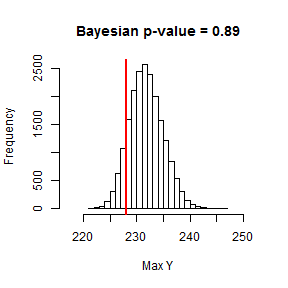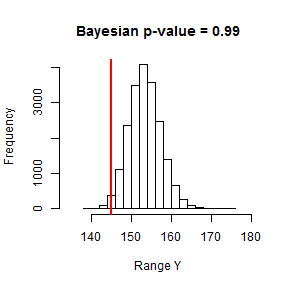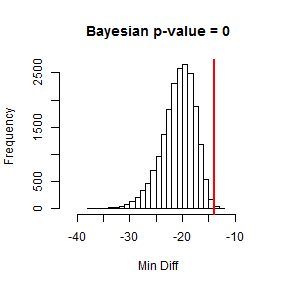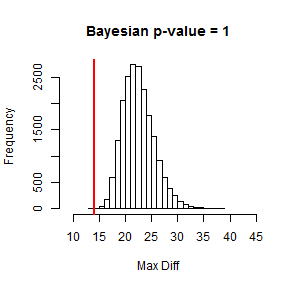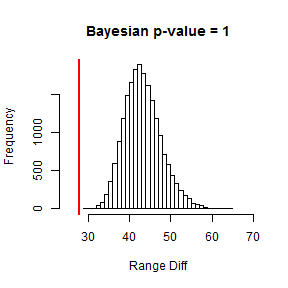Many of the Bayesian p-values are near zero or one so the model does not appear to fit the data well. It might be better to perform the analysis on the log scale.